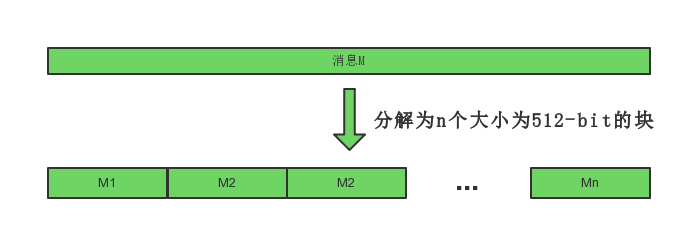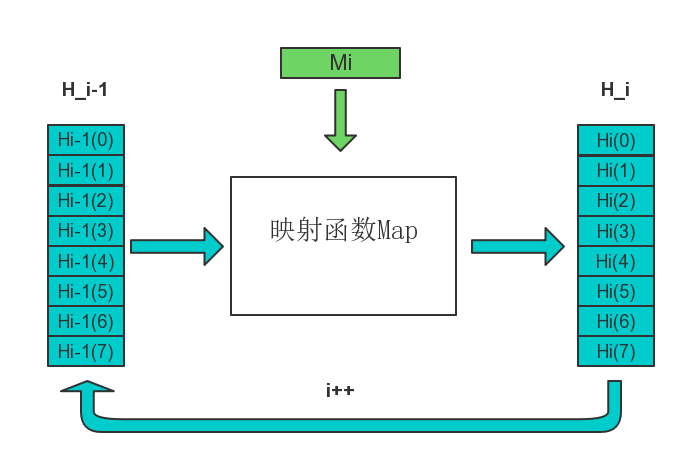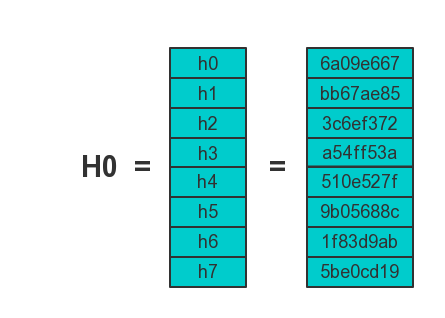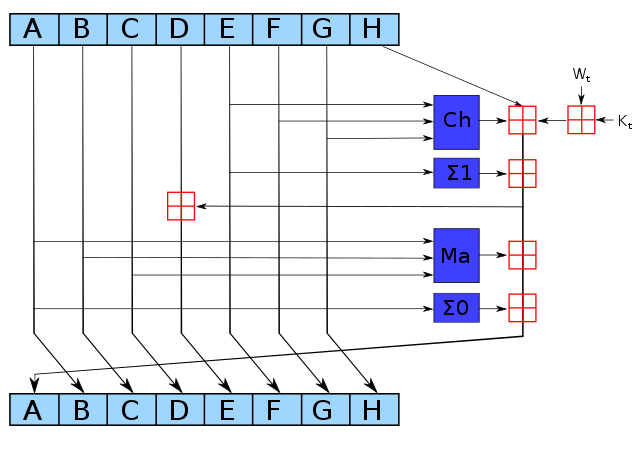# SHA256算法原理详解

## 1. SHA256简介

SHA256是SHA-2下细分出的一种算法

SHA-2，名称来自于安全散列算法2（英语：Secure Hash Algorithm 2）的缩写，一种密码散列函数算法标准，由美国国家安全局研发，属于SHA算法之一，是SHA-1的后继者。

SHA-2下又可再分为六个不同的算法标准

干他100天成为区块链程序员，红军大叔带领着我们，fighting!

A7FCFC6B5269BDCCE571798D618EA219A68B96CB87A0E21080C2E758D23E4CE9

## 2. SHA256原理详解

### 2.1 常量初始化

SHA256算法中用到了8个哈希初值以及64个哈希常量

h0 := 0x6a09e667
h1 := 0xbb67ae85
h2 := 0x3c6ef372
h3 := 0xa54ff53a
h4 := 0x510e527f
h5 := 0x9b05688c
h6 := 0x1f83d9ab
h7 := 0x5be0cd19


$0.414213562373095048 \approx 6*16^{-1} + a*16^{-2} + 0*16^{-3} + ...$

428a2f98 71374491 b5c0fbcf e9b5dba5
3956c25b 59f111f1 923f82a4 ab1c5ed5
d807aa98 12835b01 243185be 550c7dc3
72be5d74 80deb1fe 9bdc06a7 c19bf174
e49b69c1 efbe4786 0fc19dc6 240ca1cc
2de92c6f 4a7484aa 5cb0a9dc 76f988da
983e5152 a831c66d b00327c8 bf597fc7
c6e00bf3 d5a79147 06ca6351 14292967
27b70a85 2e1b2138 4d2c6dfc 53380d13
650a7354 766a0abb 81c2c92e 92722c85
a2bfe8a1 a81a664b c24b8b70 c76c51a3
d192e819 d6990624 f40e3585 106aa070
19a4c116 1e376c08 2748774c 34b0bcb5
391c0cb3 4ed8aa4a 5b9cca4f 682e6ff3
748f82ee 78a5636f 84c87814 8cc70208
90befffa a4506ceb bef9a3f7 c67178f2


### 2.2 信息预处理(pre-processing)

SHA256算法中的预处理就是在想要Hash的消息后面补充需要的信息，使整个消息满足指定的结构。

STEP1：附加填充比特

：以信息“abc”为例显示补位的过程。

a,b,c对应的ASCII码分别是97,98,99

61626380 00000000 00000000 00000000
00000000 00000000 00000000 00000000
00000000 00000000 00000000 00000000
00000000 00000000


STEP2：附加长度值

wiki百科中给出的原文是：append length of message (before pre-processing), in bits, as 64-bit big-endian integer

SHA256用一个64位的数据来表示原始消息的长度。

61626380 00000000 00000000 00000000
00000000 00000000 00000000 00000000
00000000 00000000 00000000 00000000
00000000 00000000 00000000 00000018


### 2.3 逻辑运算

SHA256散列函数中涉及的操作全部是逻辑的位运算

$Ch(x,y,z) = (x \land y) \oplus (\neg x \land z)$
$Ma(x,y,z) = (x \land y) \oplus (x \land z) \oplus (y \land z)$
$\Sigma_{0}(x) = S^{2}(x) \oplus S^{13}(x) \oplus S^{22}(x)$
$\Sigma_{1}(x) = S^{6}(x) \oplus S^{11}(x) \oplus S^{25}(x)$
$\sigma_{0}(x) = S^{7}(x) \oplus S^{18}(x) \oplus R^{3}(x)$
$\sigma_{1}(x) = S^{17}(x) \oplus S^{19}(x) \oplus R^{10}(x)$

$\land$ 按位“与”
$\neg$ 按位“补”
$\oplus$ 按位“异或”
$S^{n}$ 循环右移n个bit
$R^{n}$ 右移n个bit

### 2.4 计算消息摘要

(break message into 512-bit chunks)H1经过第二个数据块得到H2，……，依次处理，最后得到Hn，Hn即为最终的256-bit消息摘要STEP1：构造64个字（word）

break chunk into sixteen 32-bit big-endian words w, …, w

$W_{t} = \sigma_{1}(W_{t-2}) + W_{t-7} + \sigma_{0}(W_{t-15}) + W_{t-16}$

STEP2：进行64次循环ABCDEFGH一开始的初始值分别为$H_{i-1}(0),H_{i-1}(1),…,H_{i-1}(7)$

Kt是第t个密钥，对应我们上文提到的64个常量

Wt是本区块产生第t个word。原消息被切成固定长度512-bit的区块，对每一个区块，产生64个word，通过重复运行循环n次对ABCDEFGH这八个字循环加密。

## 3. SHA256算法伪代码

Note: All variables are unsigned 32 bits and wrap modulo 232 when calculating

Initialize variables
(first 32 bits of the fractional parts of the square roots of the first 8 primes 2..19):
h0 := 0x6a09e667
h1 := 0xbb67ae85
h2 := 0x3c6ef372
h3 := 0xa54ff53a
h4 := 0x510e527f
h5 := 0x9b05688c
h6 := 0x1f83d9ab
h7 := 0x5be0cd19

Initialize table of round constants
(first 32 bits of the fractional parts of the cube roots of the first 64 primes 2..311):
k[0..63] :=
0x428a2f98, 0x71374491, 0xb5c0fbcf, 0xe9b5dba5, 0x3956c25b, 0x59f111f1, 0x923f82a4, 0xab1c5ed5,
0xd807aa98, 0x12835b01, 0x243185be, 0x550c7dc3, 0x72be5d74, 0x80deb1fe, 0x9bdc06a7, 0xc19bf174,
0xe49b69c1, 0xefbe4786, 0x0fc19dc6, 0x240ca1cc, 0x2de92c6f, 0x4a7484aa, 0x5cb0a9dc, 0x76f988da,
0x983e5152, 0xa831c66d, 0xb00327c8, 0xbf597fc7, 0xc6e00bf3, 0xd5a79147, 0x06ca6351, 0x14292967,
0x27b70a85, 0x2e1b2138, 0x4d2c6dfc, 0x53380d13, 0x650a7354, 0x766a0abb, 0x81c2c92e, 0x92722c85,
0xa2bfe8a1, 0xa81a664b, 0xc24b8b70, 0xc76c51a3, 0xd192e819, 0xd6990624, 0xf40e3585, 0x106aa070,
0x19a4c116, 0x1e376c08, 0x2748774c, 0x34b0bcb5, 0x391c0cb3, 0x4ed8aa4a, 0x5b9cca4f, 0x682e6ff3,
0x748f82ee, 0x78a5636f, 0x84c87814, 0x8cc70208, 0x90befffa, 0xa4506ceb, 0xbef9a3f7, 0xc67178f2

Pre-processing:
append the bit '1' to the message
append k bits '0', where k is the minimum number >= 0 such that the resulting message
length (in bits) is congruent to 448(mod 512)
append length of message (before pre-processing), in bits, as 64-bit big-endian integer

Process the message in successive 512-bit chunks:
break message into 512-bit chunks
for each chunk
break chunk into sixteen 32-bit big-endian words w[0..15]

Extend the sixteen 32-bit words into sixty-four 32-bit words:
for i from 16 to 63
s0 := (w[i-15] rightrotate 7) xor (w[i-15] rightrotate 18) xor(w[i-15] rightshift 3)
s1 := (w[i-2] rightrotate 17) xor (w[i-2] rightrotate 19) xor(w[i-2] rightshift 10)
w[i] := w[i-16] + s0 + w[i-7] + s1

Initialize hash value for this chunk:
a := h0
b := h1
c := h2
d := h3
e := h4
f := h5
g := h6
h := h7

Main loop:
for i from 0 to 63
s0 := (a rightrotate 2) xor (a rightrotate 13) xor(a rightrotate 22)
maj := (a and b) xor (a and c) xor(b and c)
t2 := s0 + maj
s1 := (e rightrotate 6) xor (e rightrotate 11) xor(e rightrotate 25)
ch := (e and f) xor ((not e) and g)
t1 := h + s1 + ch + k[i] + w[i]
h := g
g := f
f := e
e := d + t1
d := c
c := b
b := a
a := t1 + t2

Add this chunk's hash to result so far:
h0 := h0 + a
h1 := h1 + b
h2 := h2 + c
h3 := h3 + d
h4 := h4 + e
h5 := h5 + f
h6 := h6 + g
h7 := h7 + h

Produce the final hash value (big-endian):
digest = hash = h0 append h1 append h2 append h3 append h4 append h5 append h6 append h7


SHA-2 wiki

SHA-256算法实现

# 知识填补

## 大端和小端（Big endian and Little endian）

Big endian 认为第一个字节是最高位字节（按照从低地址到高地址的顺序存放数据的高位字节到低位字节）

0x0000 0x12
0x0001 0x34
0x0002 0xab
0x0003 0xcd

big-Big_endian 0x12 0x34 0xab 0xcd
little-endian 0xcd 0xab 0x34 0x12

08-093051

#### SHA256算法原理详解图

05-269822

#### MD5和SHA256加密04-101万+

#### 如何用Sha256进行简单的加密或者解密

01-223948

#### SHA-256摘要算法（带示例）

06-293449

#### SHA-256算法实现过程

02-101万+

#### SHA算法原理

04-019349

#### SHA算法系列介绍

05-222859

#### SHA1和SHA256算法C语言实现

11-247876

#### HmacSHA256

07-311万+

#### SHA1校验算法C语言实现©️2020 CSDN 皮肤主题: 像素格子 设计师: CSDN官方博客点击重新获取扫码支付1.余额是钱包充值的虚拟货币，按照1:1的比例进行支付金额的抵扣。
2.余额无法直接购买下载，可以购买VIP、C币套餐、付费专栏及课程。余额充值